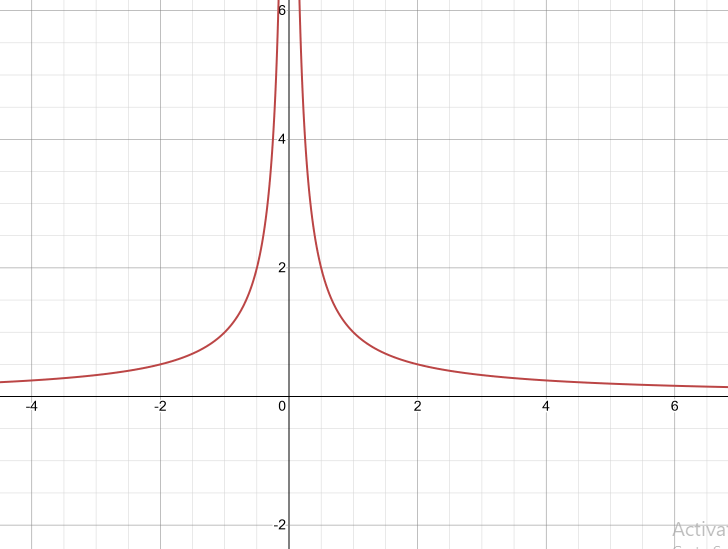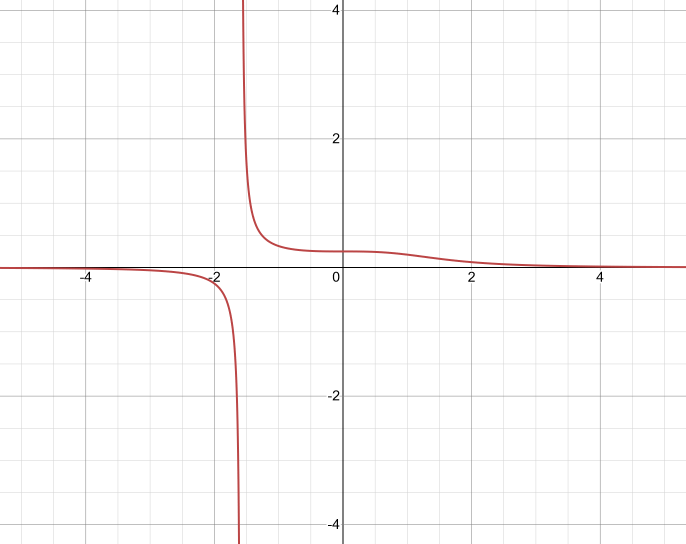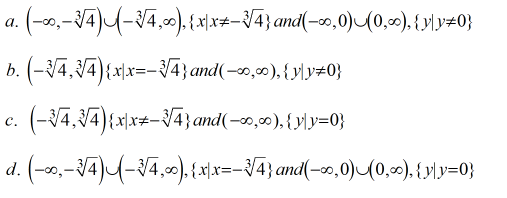Domain and Range homework Help at TutorEye

# Best Homework Help For Domain and Range

## Domain and Range:

The domain of a function is the set of all the function's potential inputs.A domain is a collection of "all the values" that make up a function.Example, considered a function f(x) = 3x, then the domain is the all the possible values of   x = {1,2,3,..}.

The set of all the outputs of a function is its range.

Pre-images are domain items, whereas images are mapped co-domain(range) components.

In the above example, the range is {3,6,9,12,...}, all the possible output of function f(x).

## Domain and Range Sample Questions:

Question 1: Find the domain of f(x),

f(x) = 2x^2 + 4x + 2

(a) (-∞, +∞)

(b) (+2, -2)

(c) (-2, +2)

(d) (-2, -2)

Explanation: Here, {x|x∈R}

Question 2: Find the domain of f(x),

f(x)= 1/√x-5

(a) (-∞, +∞)

(b) (-∞, 5)

(c) (5, -∞)

(d) (5, ∞)

Explanation: Value of x is always greater than 5, x>5, x not equal to 5.

Question 3: f(x) = x^2 + 2

g(x) = √x-4

Find the domain of (fOg)(x)?

(a) (-4, +∞)

(b) (4, ∞)

(c) (-∞, 4)

(d) (∞, -4)

Explanation: We conclude that the value of x starts from 4 and goes to  infinity.

Question 4: Find the domain of f(g(x)),

f(x) =√x-4, g(x) = 3/(x - 9)

(a) (9, 21/2)

(b) (9, -21/2)

(c) (-9, 21/2)

(d) (-9, -21/2)

Explanation: Value of x: {x | 9 < x ≤ 21/2}

Question 5: Find the domain of f(g(x))

f(x) =3x - 4, g(x) = √x+2

(a) (-2, 2)

(b) (-2, ∞)

(c) (-∞, ∞)

(d) (-∞, 2)

Explanation: Value of x: {x | x ≥ -2}

Question 6: Find the range of f(x)

f(x) = 2x^2 + 4x + 2

(a) (0, -∞)

(b) (-∞, 0)

(c) (0, ∞)

(d) (-∞, ∞)

Explanation: {y | y ≥ 0}

Question 7: Find the range of f(x)

f(x) = 1/√x-5

(a) (0, ∞)

(b) (-∞, 0)

(c) (0, -∞)

(d) (-∞, ∞)

Explanation: {y | y > 0}

Question 8: Find the range of the function f(x) from the graph?(a) (0, ∞), {y | y > 0}

(b) (-∞, 0), {y | y > 0}

(c) (0, ∞), {y | y ≥ 0}

(d) (-∞, 0), {y | y ≥ 0}

Explanation: Get it resolve from the Graph.

Question 9: Find the domain of the function f(x) from the graph?(a) (-∞, ∞), {x | x ≠ 0}

(b) (-∞, 0)U(0, ∞), {x | x ≠ 0}

(c) (-∞, 0)U(0, ∞), {x | x = 0}

(d) (-∞, ∞), {x | x = 0}

Explanation: Get it resolve from the Graph.

Question 10: Find the domain and range of the function from the graph?